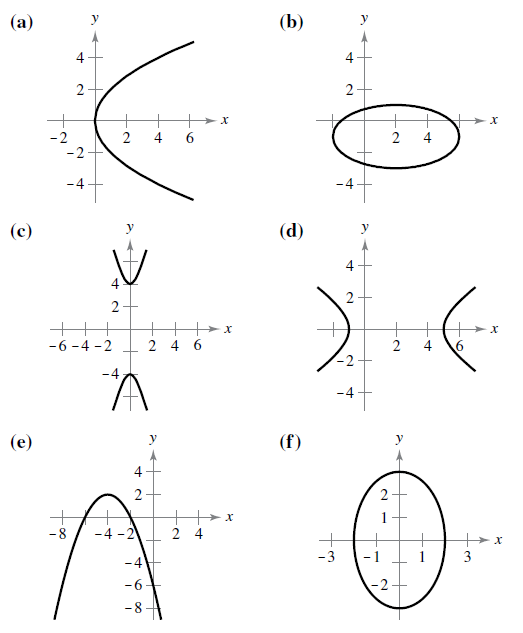Chapter 10.1, Problem 4E

Chapter
Section
Textbook Problem

# Matching In Exercises 5-10, match the equation with its graph. [The graphs are labeled (a), (b), (c), (d), (e). and (f).]( x − 2 ) 2 16 + ( y + 1 ) 2 4 = 1

To determine
The correct match of the equation (x2)216+(y+1)24=1  with its corresponding graph.

Explanation

Given: The equation (x2)216+(y+1)24=1  and the graph of equations are,

Calculation: Consider the equation (x2)216+(y+1)24=1 . Since the given equation is of the type (xh)2a2+(yk)2b2=1 which is the standard form of an ellipse and the ellipse has a center at (h,k) that (2,1) with the semi-major axis a=4 and semi minor axis b=2

### Still sussing out bartleby?

Check out a sample textbook solution.

See a sample solution

#### The Solution to Your Study Problems

Bartleby provides explanations to thousands of textbook problems written by our experts, many with advanced degrees!

Get Started